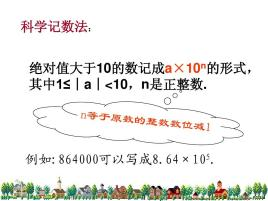Home Technology Scientific notation

# Scientific notation

## Concept

Scientific notation is a method of counting numbers. Express a number in the form of multiplying a and 10 to the nth power (1≤|a|<10, a is not a fraction, n is an integer), this notation is called scientific notation. For example: 19971400000000=1.99714×10^13. Calculators or computers express the power of 10 by using E or e, which is 1.99714E13=19971400000000.

## Notation and benefits

When we want to mark or calculate a larger or smaller one with more digits, use scientific notation to avoid wasting a lot of space and time.

### Form

The form of scientific notation is composed of the product of two numbers. Expressed as a×10^b(aEb)

One of the factors is a (1≤|a|<10), and the other factor is 10^n.

### Convenient

When expressing numbers in scientific notation, the sign of the number is not changed, only the writing form of the number is changed, which can conveniently express some extremes encountered in daily life. Large or very small number. For example, the speed of light is about 300,000,000 meters per second; the population of the world is about 6,100,000,000.

Such a number is very inconvenient to read and write. We can avoid writing so many repeated 0s. , Express it in this form: 6,100,000,000=6.1×10^9,

or: 0.00001=1×10^-5, that is, the number whose absolute value is less than 1 can also be expressed in scientific notation It is in the form of a times 10 to the power of negative n.

### Notation

If |x|>1, it is recorded in the form of, the value of is determined by the number of digits, and m is the number of digits of x, then,

If |x|<1, then,, where m is the number of digits of x and m₁ is the number of significant digits of x.

## Accuracy

Use scientific notation to a×10^n number, its accuracy is based on the digit of the last number of a in the original number.

Such as:

13600, accurate to the tenth place, recorded as: 1.360X10^4

13200, accurate to the hundredth place, recorded as: 1.32X10^ 4

322000, accurate to thousands, written as: 3.22X10^5

## Operation

Related expression form

aEb= a×10^b

(1) 3×10^4+4×10^4=7×10^4

ie aEc±bEc=﹙a±b﹚Ec

(2) 3E6×6E5=18E11=1.8E12

ie aEM×bEN=abE(M+N)

(3)-6E4÷3E3 =-2E1that is, aEM÷bEN=a/bE(MN)⑷

Some related derivations

(aEc)^2=(aEc)( aEc)=a^2E2c

(aEc)^3=(aEc)(aEc)(aEc)=a^3E3c

(aEc)^n=a^nEnc p>

a×10^logb=ab

aElogb=ab

(1) n"E" formula 3E4E5=30000E5=3E9

aEbEc=aEb+c

6E-3E-6E3=0.006E-6E3

=0.000000006E3

=6E-6

That is, aEbEcEd=aEb+c+d

to get aEa1Ea2Ea3.......Ean=aEa1+a2+a3+.......+an

(2) n"E" formula and sequence

According to n"E" formula aEa1Ea2Ea3.......Ean=aEa1+a2+a3+.......+an

Get aESn

The arithmetic n terms and formula na1+n(n+1)/2×d

aEna1+n(n+1)/2×d

Ratio n term and formula Sn=a1n(q=1) or n(1-q^n)/1-q

aESn [Sn=a1n(q=1) or n (1-q^n)/1-q(q≠1) ]

Counting the general terms of the sequence

The arithmetic difference: aEan=aEa1+(n-1)d

p>

Ratio: aEan=aEa1q^n-1

(3) aEb and aE-b

aEb=a×10^b

aE-b=a×10^-b Positive and negative b determines the direction of E

Significance of scientific notation

"aE" means that it does not have the meaning of scientific notation, and aE=a

"Ea" means that it has the meaning of scientific notation, that is, when Ea=1Ea a=3, 1E3=1000

aEb=ca=c/Eb

## Settings

Set the scientific notation format in Excel

In Microsoft Excel software, you can set the numeric data in the cell Set to scientific notation format, and take Excel 2010 as an example to introduce the setting method:

Step 1, open the Excel2010 worksheet window, and select the cell that needs to set the scientific notation format. Right-click the selected cell and select the "Format Cell" command in the shortcut menu that opens.

Step 2, in the opened Excel2010 "Format Cells" dialog box, switch to the "Number" tab. Select the "Scientific Notation" option in the "Classification" list, and set the number of decimal places in the "Decimal Places" spin box on the right. After setting, click the "OK" button.

This article is from the network, does not represent the position of this station. Please indicate the origin of reprint